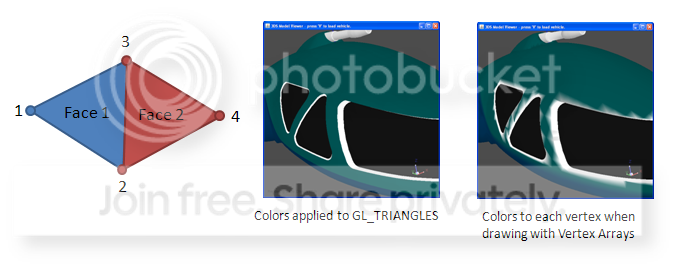# ? Colors per vertex or per face ?

I’m trying to render a 3DS model and I’m having trouble rendering properly using Vertex Arrays - specifically getting the colors correct. This is sort of a followup to another topic I had posted: Texturing many triangles - 3DS loader question

Because I load a 3DS model that could be composed of multiple textures/materials I had to sort my faces by material and then group them into separate buckets. When I render using immediate mode I perform the following:

``````
// Loop over my buckets/groups of faces
for (i=0; i<groups.size(); i++) {
// Set the color of all of this groups triangles based on
// color of this bucket.
glColor3d(groups[i].materialcolor);

glBegin(GL_TRIANGLES);
// Loop over the faces in each group
for (j=0; j<groups[i].size(); j++) {
glNormal(..)
glVertex3d(groups[i].getFace(j).firstVertex);
glVertex3d(groups[i].getFace(j).secondVertex);
glVertex3d(groups[i].getFace(j).thirdVertex);
}
glEnd();
}

``````

Great, that works well. The faces are draw with the correct colors and between triangles of different colors I have nice crisp divisions. Now, when I render using Vertex Arrays I have to create an array of vertices, of normals, and of colors. But the color data (material color) that I get is obtained from the faces. So effectively I loop over each face and assign the color/material for that face to each of its 3 vertices.

The problem is that if a neighbor face has a different color/material and it is processed next, then the 2 shared vertices will now get the color of that second face. So now when you render the first triangle and apply the color to its three vertices then it will have a blend between two colors. The result will look like you can see in the far right image…the colors “bleed” together rather than being nice and crisp.(Link to picture)

The way I generate that second picture is to render using Vertex Arrays in this way:

``````
glEnableClientState(GL_NORMAL_ARRAY);
glEnableClientState(GL_VERTEX_ARRAY);
glEnableClientState(GL_COLOR_ARRAY);

glNormalPointer(GL_FLOAT, 0, normals.position(0));
glVertexPointer(3, GL_FLOAT, 0, vertices.position(0));
glColorPointer(3, GL_FLOAT, 0, colors.position(0));

// Loop over my buckets/groups of faces. The 'indices' array stores the
// ids of the vertices of each face.
for (i=0; i<groups.size(); i++) {
glDrawElements(GL_TRIANGLES,
groups[i].length * 3,
GL_UNSIGNED_INT,
indices.position(groups[i].offset * 3));

}

glDisableClientState(GL_NORMAL_ARRAY);
glDisableClientState(GL_VERTEX_ARRAY);
glDisableClientState(GL_COLOR_ARRAY);

``````

So if I use glColorPointer() as I do above, the solution is wrong because sometimes the triangles that get rendered have the wrong color (i.e. they have their neighbor’s color) and so you see that bleed through.

Is there another way I should be doing this rendering? Since I know the color/material of each face, should I move instead use some glColor3() method inside the for-loop (i.e. before the glDrawElements() call)?

You need to duplicate each vertex if it is used with different attributes. The case you describe is one of these. Typically vertex positions stay the same but any of the other attributes (normal, color, texture coordinates) can be different for each face. In all these cases you basically have to create a copy of the vertex, one for each face.

thanks…that makes sense though it seems kind of costly to duplicate the vertices.

I tried my idea of setting the color within each ‘group’ call (in the for loop) without using the glColorPointer(). That had better results though I still saw some bad triangles near the borders between materials. It was kind of unexpected but it might be some other setting of mine that is the cause - not sure.